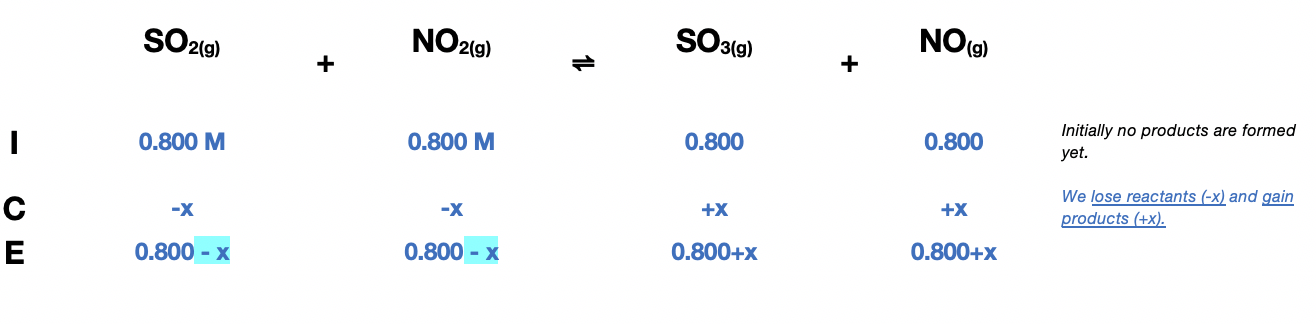# Problem: At a particular temperature, K = 3.75 for the reaction SO2(g) + NO2(g) ⇌ SO3(g) + NO(g)If all four gases had initial concentrations of 0.800 M, calculate the equilibrium concentrations of the gases.

###### FREE Expert Solution

Constructing an ICE Chart, we have:$\overline{){{\mathbf{K}}}_{{\mathbf{c}}}{\mathbf{=}}\frac{\mathbf{products}}{\mathbf{reactants}}{\mathbf{=}}\frac{\left[{\mathrm{SO}}_{3}\right]\left[\mathrm{NO}\right]}{\left[{\mathrm{SO}}_{2}\right]\left[{\mathrm{NO}}_{2}\right]}}$

Solve for x:

${\mathbf{3}}{\mathbf{.}}{\mathbf{75}}{\mathbf{=}}\frac{\left(0.800+x\right)\left(0.800+x\right)}{\mathbf{\left(}\mathbf{0}\mathbf{.}\mathbf{800}\mathbf{-}\mathbf{x}\mathbf{\right)}\mathbf{\left(}\mathbf{0}\mathbf{.}\mathbf{800}\mathbf{-}\mathbf{x}\mathbf{\right)}}\phantom{\rule{0ex}{0ex}}\phantom{\rule{0ex}{0ex}}{\mathbf{3}}{\mathbf{.}}{\mathbf{75}}{\mathbf{=}}\frac{{\mathbf{\left(}\mathbf{0}\mathbf{.}\mathbf{800}\mathbf{+}\mathbf{x}\mathbf{\right)}}^{\mathbf{2}}}{{\mathbf{\left(}\mathbf{0}\mathbf{.}\mathbf{800}\mathbf{-}\mathbf{x}\mathbf{\right)}}^{\mathbf{2}}}\phantom{\rule{0ex}{0ex}}\phantom{\rule{0ex}{0ex}}\sqrt{\mathbf{3}\mathbf{.}\mathbf{75}}{\mathbf{=}}\sqrt{\frac{{\mathbf{\left(}\mathbf{0}\mathbf{.}\mathbf{800}\mathbf{+}\mathbf{x}\mathbf{\right)}}^{\mathbf{2}}}{{\mathbf{\left(}\mathbf{0}\mathbf{.}\mathbf{800}\mathbf{-}\mathbf{x}\mathbf{\right)}}^{\mathbf{2}}}}\phantom{\rule{0ex}{0ex}}\phantom{\rule{0ex}{0ex}}{\mathbf{1}}{\mathbf{.}}{\mathbf{94}}{\mathbf{=}}\frac{\mathbf{0}\mathbf{.}\mathbf{800}\mathbf{+}\mathbf{x}}{\mathbf{0}\mathbf{.}\mathbf{800}\mathbf{-}\mathbf{x}}\phantom{\rule{0ex}{0ex}}\phantom{\rule{0ex}{0ex}}\left(1.94\right)\left(0.800-x\right){\mathbf{=}}{\mathbf{0}}{\mathbf{.}}{\mathbf{800}}{\mathbf{+}}{\mathbf{x}}$

79% (301 ratings)###### Problem Details

At a particular temperature, K = 3.75 for the reaction

SO2(g) + NO2(g) ⇌ SO3(g) + NO(g)

If all four gases had initial concentrations of 0.800 M, calculate the equilibrium concentrations of the gases.

Frequently Asked Questions

What scientific concept do you need to know in order to solve this problem?

Our tutors have indicated that to solve this problem you will need to apply the The Reaction Quotient concept. You can view video lessons to learn The Reaction Quotient. Or if you need more The Reaction Quotient practice, you can also practice The Reaction Quotient practice problems.

What professor is this problem relevant for?

Based on our data, we think this problem is relevant for Professor Orr's class at NYU.

What textbook is this problem found in?

Our data indicates that this problem or a close variation was asked in Chemistry: An Atoms First Approach - Zumdahl Atoms 1st 2nd Edition. You can also practice Chemistry: An Atoms First Approach - Zumdahl Atoms 1st 2nd Edition practice problems.